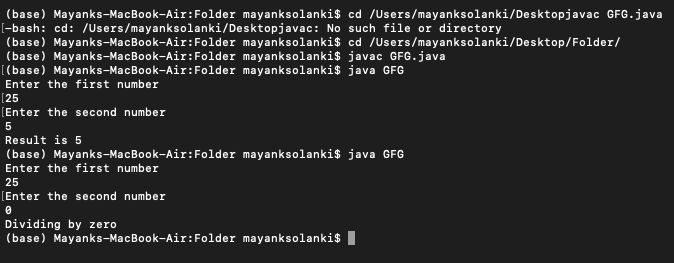Related Articles

# Java Program to use Catch to Handle the Exception

• Last Updated : 11 Nov, 2020

The exception is the event occurs when the program is executing. Due to this exception, the normal flow of the program will get disrupts. Whenever an exception occurs in the method, the method creates an object and sends that object to the runtime system. For example, the file needs to be open is not found, class not found exception, Arithmetic Exception, SQL Exception, etc.

To handle these exceptions there are 4 standard techniques:

• Try-catch
• Throw
• Throws
• Using HandlerExceptionResolver interface (Only in Spring-framework)

Catch usage is described here below:

Syntax:

```try {

// Put the code in the try block which may occur any
// kind of exception
}
catch (ExceptionName e) {

// handle the exception
}

```

There are two examples discussed below:

1. Using predefined exception Class
2. Using defining own exception Class

Example 1: Usage of catch with a predefined exception class

This is an example of a predefined exception which is handled by try and catch block.

## Java

 `// Importing Classes/Files``import` `java.io.*;``import` `java.util.*;`` ` `// Importing Scanner Class for user input``import` `java.util.Scanner;`` ` `class` `GFG {``   ` `    ``// Main driver method``    ``public` `static` `void` `main(String[] args)``    ``{``        ``// Taking input from user``        ``Scanner input = ``new` `Scanner(System.in);`` ` `        ``// Taking input of first number``        ``System.out.println(``"Enter the first number"``);``        ``Integer number1 = input.nextInt();`` ` `        ``// Taking input of second number``        ``System.out.println(``"Enter the second number"``);``        ``Integer number2 = input.nextInt();`` ` `        ``// Considering values in order to show execution``        ``// number1 = 30; number2 = 10;`` ` `        ``try` `{`` ` `            ``// Dividing both the numbers``            ``int` `result = number1 / number2;`` ` `            ``// If number2 = 0, method will create the object``            ``// of ArithmeticException class and throw it``            ``System.out.println(``"Result is "` `+ result);``            ``// Printing the result``        ``}`` ` `        ``// If there is any  exception in the try block``        ``// then catch will handle the exception``        ``catch` `(ArithmeticException e) {`` ` `            ``// Message printed after catching the exception``            ``System.out.println(``"Dividing by zero"``);``        ``}``    ``}``}`

Output:Example 2: Usage of catch by defining the exception class

In this example, the exception is user-defined in which exception class is written as follows:

## Java

 `// Java Program to Use catch to handle the exception`` ` `// Importing generic Classes/Files``import` `java.io.*;``import` `java.util.*;`` ` `class` `GFG {`` ` `    ``// Main driver method``    ``public` `static` `void` `main(String[] args)``    ``{`` ` `        ``// Taking input from keyboard/user``        ``Scanner input = ``new` `Scanner(System.in);`` ` `        ``// Enter any name``        ``System.out.println(``"Enter the name"``);``        ``String name = input.next();``        ``try` `{``            ``// Enter age``            ``System.out.println(``"Enter the age"``);`` ` `            ``// Using nextInt function to read integer``            ``Integer age = input.nextInt();``            ``if` `(age <= ``18``) {``                ``MyException me = ``new` `MyException();``                ``throw` `me;``                ``// If age is less then equal to 18 then the``                ``// object of MyException class will be throw``                ``// here``            ``}``            ``else`` ` `            ``// If age is greater then 18 i.e no exception is``            ``// there then try block will execute``            ``{``                ``System.out.println(``"name:"` `+ name);``                ``System.out.println(``"age:"` `+ age);``            ``}``        ``}``        ``catch` `(MyException e)``        ``// If the exception will occur then the object that``        ``// throw in the try block will be copied in to this``        ``// object``        ``{``            ``System.out.println(e);``        ``}``    ``}``}``// User defined MyException class``class` `MyException ``extends` `RuntimeException {``    ``public` `String toString()``    ``{``        ``// toString method will get Override here``        ``return` `"Age must be greater then 18"``;``    ``}``}`

```Input 1:
Enter the name : Geek
Enter the age: 18

Output:
Age must be greater then 18  // Age is equal to 18 so the exception is occured

Input 2:
Enter the name : Geek
Enter the age: 20

Output:
name: Geek
age:20                      // Age is greater then 18 so no exception.
```

Attention reader! Don’t stop learning now. Get hold of all the important Java Foundation and Collections concepts with the Fundamentals of Java and Java Collections Course at a student-friendly price and become industry ready. To complete your preparation from learning a language to DS Algo and many more,  please refer Complete Interview Preparation Course.

My Personal Notes arrow_drop_up